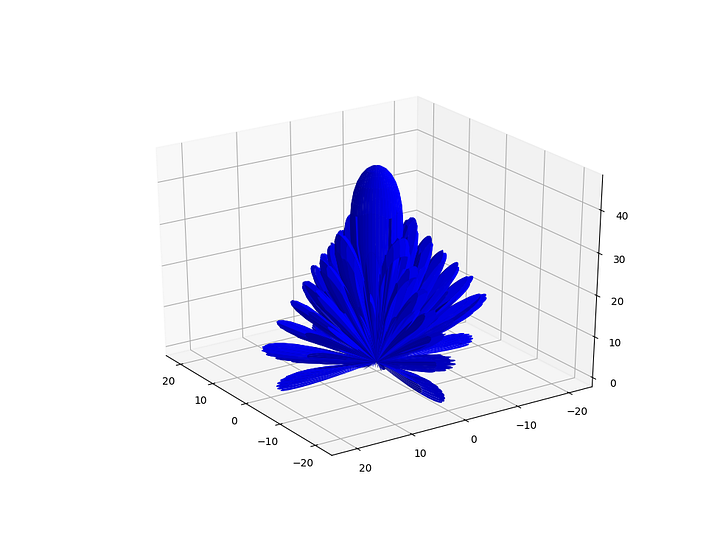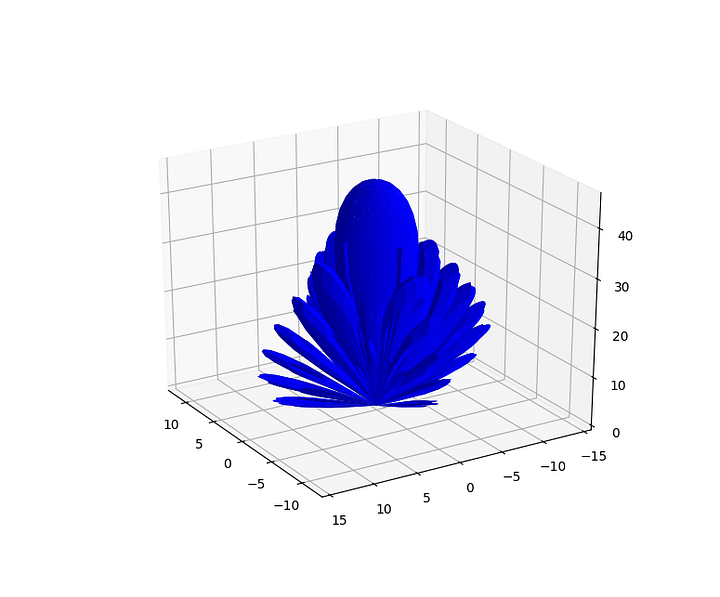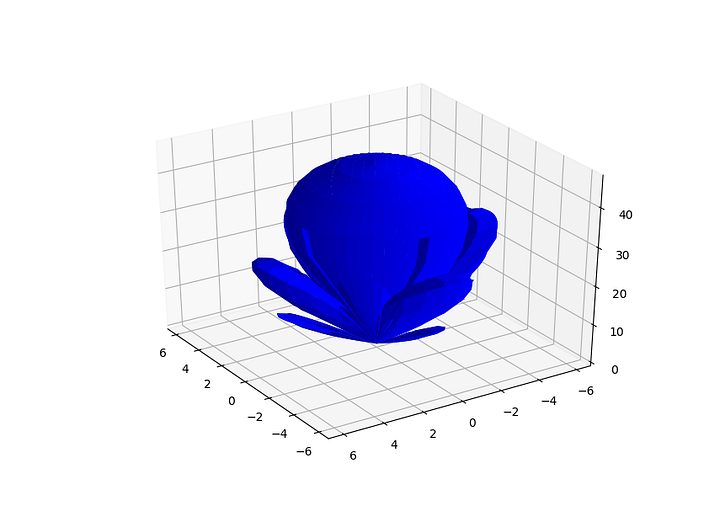# Antenna Arrays And Python – The Array (finally!)

As mentioned in my intro post an array antenna is a set of individual antennas connected to work as a single antenna. So far we’ve covered the individual antennas, i.e. the square patches, now it’s time to look at how they can be connected to work together.

### Array Factor Fun

You can’t get far digging into arrays before you come across the Array Factor. It looks complicated but to me the easiest way to think of it is that it combines every elements position, radiating amplitude and radiating phase to give the overall array performance.

The Array Factor demonstrates that by altering an element, such as its position or phase, we can alter the arrays properties. For example the arrays beam could be steered to a desired position by altering the phase of each element.

The Array Factor is given by:

Where:

θ, φ = Direction from origin

N = number of elements

An= Amp of element

βn = Phase of element in rads

k0 = 2π/λ rads/m

And:

is the relative phase of incident wave at element n located at xn, yn, zn.

#### Array Factor in Python

The script below shows how easy it is to calculate the Array Factor in Python:

### Array Radiation Pattern, Directivity & Gain

The above Array Factor equation is independent of each elements individual radiating pattern. The overall radiation pattern of an array is determined by the array factor combined with the radiation pattern of each element, Fn(θn, φn), giving:

The overall radiation pattern results in a certain directivity and thus gain linked through the efficiency as discussed previously.

### Some Examples

To demonstrate the effects the individual element patterns have on the overall array performance we can investigate some examples using Python.

#### Isotropic antenna elements

In this case each element radiates equally in all directions so Fn(θn, φn) is the same for each θn, φn. The antenna radiation pattern is now just the Array Factor as described in the code above.15×15 Array of Isotropic Elements

#### Patch antenna elements

Using elements described by the PatchFunction discussed previously:

`PatchFunction(θ, φ, Freq, 10.7e-3, 10.47e-3, 3e-3, 2.5)`15×15 Array of patches

Python script for patch array:

#### Horn antenna elements

And finally using horn antenna elements represented by cos²⁸(θ) function.15×15 Array of cos²⁸(θ) Horns

Python script for horn array:

Next time we can see how to calculate an elements phase to steer the beam.